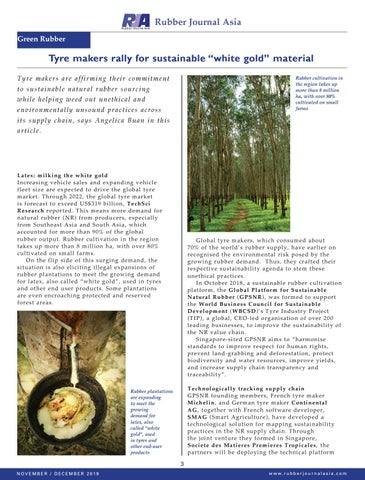__MAIN_TEXT__Rubber Journal Asia Green Rubber

Tyre makers rally for sustainable “white gold” material Tyre makers are affirming their commitment

Rubber cultivation in the region takes up more than 8 million ha, with over 80% cultivated on small farms

to sustainable natural rubber sourcing while helping weed out unethical and

environmentally unsound practices across

Lat e x : mi l k i n g t h e wh ite g o ld Inc r e a s i n g v e h i cl e s a l e s a n d e x p a n d i n g v e hicle flee t s i z e a r e e x p e c t e d t o d r i v e t h e g l o b a l tyre m ar ke t . T h ro u gh 2 0 2 2 , t h e g l o b a l t y r e m a r k e t is fore c a st t o exce e d US \$ 3 1 9 b i l l i o n , T e c h Sc i R es e a rc h re p ort e d . T h i s m e a n s m o r e d e m and fo r natu r a l r u b b e r ( N R ) f r o m p r o d u c e r s , e s p e cially from S o u t h e a s t A s i a a n d S o u t h A s i a , w h i c h acco u n t e d f o r m o r e t h a n 9 0 % o f t h e g l o b a l rub ber ou t p u t . R u b b e r c u l t i v a t i o n i n t h e r e gio n tak e s u p m o r e t h a n 8 m i l l i o n h a , w i t h o v e r 80% cult i v a t ed o n sm a l l fa r m s . O n t h e f l i p s i d e o f t h i s s u r g i n g d e m a n d, the sit u a t i on i s a l so e l i ci t i n g i l l e g a l e x p a n s i o n s o f rub b e r p l a n t a t i o n s t o m e e t t h e g r o w i n g d emand for l a t e x , a l s o c a l l e d “ w h i t e g o l d ” , u s e d i n tyres and o t h e r e n d u s e r p r o d u c t s . S o m e p l a n t a tions are e v e n e n c r o a c h i n g p r o t e c t e d a n d r e s e r v ed fore s t a r e a s .

Global tyre makers, which consumed about 70% o f the w o rld’s rub b e r s up p ly, have ear lier on recognised the environmental risk posed by the growing rubber demand. Thus, they crafted their respective sustainability agenda to stem these une thic al p rac tic e s . In October 2018, a sustainable rubber cultivation p latfo rm, the Global Platform for Sustainable Natur al R ubber ( GPSNR ), w as fo rme d to suppor t the Wor ld B us ines s C ounc il f or Sus ta i nabl e Developm ent ( WB C SD)’s Tyre Industry Project (TIP ), a glo b al, C EO-le d o rganis atio n of over 200 le ading b us ine s s e s , to imp ro v e the s ustainability of the NR value chain. Singapore-sited GPSNR aims to “harmonise standards to improve respect for human rights, prevent land-grabbing and deforestation, protect biodiversity and water resources, improve yields, and inc re as e s up p ly c hain trans p are ncy and trac e ab ility”. T ec hnologic ally tr ac king s upply c hain GPSNR founding members, French tyre maker Mic helin, and Ge rman tyre make r Continental AG, together with French software developer, SMAG (Smart Agriculture), have developed a te c hno lo gic al s o lutio n fo r map p ing s u stainability practices in the NR supply chain. Through the jo int v e nture the y fo rme d in Singa por e, Soc iete des Matier es Pr em ier es T r opi cal es, the p artne rs w ill b e de p lo ying the te c hnical platf or m

Rubber plantations are expanding to meet the growing demand for latex, also called “white gold”, used in tyres and other end-user products

3 NOVEMBER / DECEMBER 2019

w w w. r u b b e r j o u r n a l a s i a . c o m